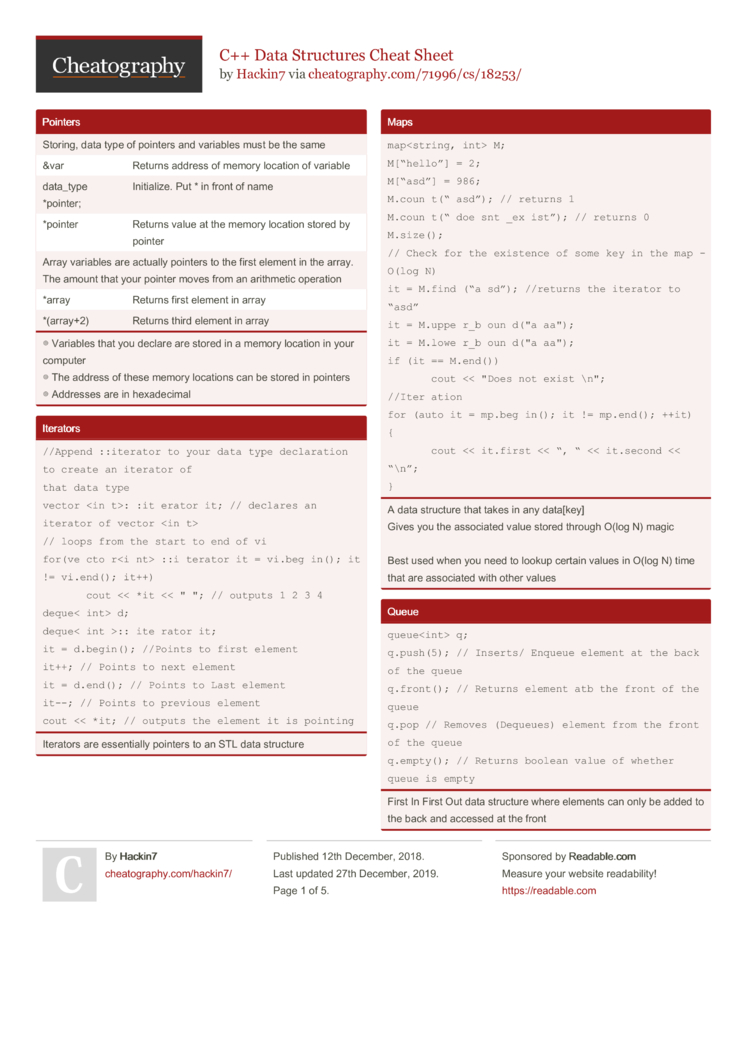C++ Data Structures Cheat Sheet by Hackin7

Pointers

 Storing, data type of pointers and variables must be the same &var Returns address of memory location of variable data_type *pointer; Initia­lize. Put * in front of name *pointer Returns value at the memory location stored by pointer Array variables are actually pointers to the first element in the array. The amount that your pointer moves from an arithmetic operation *array Returns first element in array *(array+2) Returns third element in array
◎ Variables that you declare are stored in a memory location in your
computer
◎ The address of these memory locations can be stored in pointers

Iterators

 //Append ::iterator to your data type declaration to create an iterator of that data type vector::iterator it; // declares an iterator of vector // loops from the start to end of vi for(vector::iterator it = vi.begin(); it != vi.end(); it++)     cout << *it << " "; // outputs 1 2 3 4 deque d; deque::iterator it; it = d.begin(); //Points to first element it++; // Points to next element it = d.end(); // Points to Last element it--; // Points to previous element cout << *it; // outputs the element it is pointing
Iterators are essent­ially pointers to an STL data structure

Maps

 map M; M[“hello”] = 2; M[“asd”] = 986; M.count(“asd”); // returns 1 M.count(“doesnt_exist”); // returns 0 M.size(); // Check for the existence of some key in the map - O(log N) it = M.find(“asd”); //returns the iterator to “asd” it = M.upper_bound("aaa"); it = M.lower_bound("aaa"); if (it == M.end())     cout << "Does not exist\n"; //Iteration for (auto it = mp.begin(); it != mp.end(); ++it) {     cout << it.first << “, “ << it.second << “\n”; }
A data structure that takes in any data[key]
Gives you the associated value stored through O(log N) magic

Best used when you need to lookup certain values in O(log N) time that are associated with other values

Pair

 // Initialise pair variable; // OR pair variable = make_pair(value1,value2); // Store values variable.first = value; variable second = value; // Retrieve values cout < > a; a.first = 5; a.second.first = 6; a.second.second = 7;
Stores a pair of values

Stack

 stack s; s.push(5); // push an element onto the stack - O(1) s.pop(); // pop an element from the stack - O(1) s.top(); // access the element at the top of the stack - O(1) s.empty(); // whether stack is empty - O(1)
First-­In-­Las­t-Out data structure
Only Element at the top can be accessed / removed

Vector

 // Initialize vector v; v.push_back(value); // Add element to back v.pop_back() // Remove last element v.clear(); // Remove all elements v[index] // Return element of index v.back(); // Return last element v.size(); // Return Size of vector v.empty() // Return boolean value of whether vector is empty
Like arrays but re-siz­able. You can add and remove any number of elements from any position.

Sets and Multisets

 set s; set::iterator it; multiset s; multiset::iterator it; s.insert(10); it = s.find(8) it = s.upper_bound(7); it = s.lower_bound(7); s.erase(10); //Remove element from set s.erase(it) //Can also use iterators s.empty(); s.clear(); // to loop through a set for(it = s.begin(); it != s.end(); it++)     cout << *it << " "; // outputs 2 7 10
In a set: All elements are sorted and no duplicates
Multisets can store duplicates though

Queue

 queue q; q.push(5); // Inserts/ Enqueue element at the back of the queue q.front(); // Returns element atb the front of the queue q.pop // Removes (Dequeues) element from the front of the queue q.empty(); // Returns boolean value of whether queue is empty
First In First Out data structure where elements can only be added to the back and accessed at the front

Priority Queue

 priority_queue pq; // Largest at top priority_queue, greater > pq; // Smallest at top pq.push(5); // pushes element into it. Duplicates are allowed pq.top() // Returns largest or smallest element pq.pop() // Removes largest or smallest element pq.size(); // Returns size pq.empty(); Check if queue is empty
Like a queue except that only the element with the greatest priority (eg. larges­t/s­mal­lest) can be accessed

Deque

 deque d; // access an element / modify an element (0-indexed as well) - O(1) d = 2; // change deque from {5, 10} to {2, 10} d; // returns a value of 2 d.back(); // get back (last) element - O(1) d.front(); // get front (first) element - O(1) d.clear() // Remove all elements d.push_back(5); // add an element to the back - O(1) d.push_front(10); // add an element to the front - O(1) d.pop_back(); // remove the back (last) element - O(1) d.pop_front(); // remove the front (first) element - O(1) d.size(); //Return size d.empty // Whether queue is empty
A stack and queue combined.
...or a vector that and be pushed and popped from the front as well.

Deque = Double Ended Queue!

Download the C++ Data Structures Cheat Sheet2 Pages
//media.cheatography.com/storage/thumb/hackin7_c-data-structures.750.jpg

PDF (recommended)